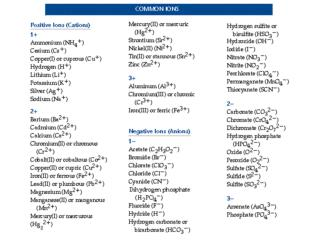Download PresentationPhysical Properties of Matter Intensive Properties : Do not change regardless of quantity.

# Physical Properties of Matter Intensive Properties : Do not change regardless of quantity.

Download Presentation## Physical Properties of Matter Intensive Properties : Do not change regardless of quantity.

- - - - - - - - - - - - - - - - - - - - - - - - - - - E N D - - - - - - - - - - - - - - - - - - - - - - - - - - -
##### Presentation Transcript

1. Physical Properties of Matter • Intensive Properties: Do not change regardless of quantity. • Examples: Density, color, chemical composition • Extensive Properties: Are dependent on quantity. • Examples: grams, milliliters, moles • ------------------------------------------------------------------------------- • Percent Yield = Theoretical Yield x 100 • Actual Yield • ------------------------------------------------------------------------------- • Molar Mass = Sum of all atomic masses in an compound or element, in grams per mole • Example: NaOH = 23+16+1 = 40 g/mol • # of particles in a mole = 6.02 x 1023 (Avogadro’s number) • ------------------------------------------------------------------------------- • Lewis Dot Structures: Only valence electrons are shown. • Examples: H• • Compound formation:

2. Combined Gas Law • P1V1 = P2V2 Where: P = pressure in atm., mmHg, torr • T1 T2 V = volume in liters, milliliters • T = temperature, Kelvin. • K = 0C + 273 • ------------------------------------------------------------------------------- • Mass number = atomic mass • = #protons + #neutrons • Atomic Number = #protons

3. Percent Composition • = molar mass of element of interest x 100 • molar mass of compound • Example: Percent composition of oxygen in water • = molar mass of oxygen in water x 100 = 16 x 100 = 89 % • molar mass of water 18 • ------------------------------------------------------------------------------- • pH • Where [H+] = molar concentration of hydrogen ion • ------------------------------------------------------------------------------- • Hydrated compounds, Nomenclature: • Name of compound • (prefix)- hydrate • Examples: • CuSO4 • 5 H2O = Copper (II) sulfate pentahydrate • FeCl3• 3H2O = Iron (III) chloride trihydrate • ------------------------------------------------------------------------------- • Oxidation: Charge increases; electrons are lost; oxygen is gained (sometimes) • Reduction: Charge decreases; electrons are gained; oxygen is lost (sometimes)